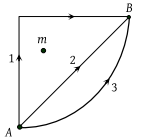The average power required to lift a 100 kg mass through a height of 50 metres in approximately 50 seconds would be

(1) 50 J/s

(2) 5000 J/s

(3) 100 J/s

(4) 980 J/s

Concept Questions :-

Power
High Yielding Test Series + Question Bank - NEET 2020

Difficulty Level:

The power of a pump, which can pump 200kg of water to a height of 200m in 10sec is (g = 10 m/s2)

(1) 40 kW

(2) 80 kW

(3) 400 kW

(4) 960 kW

Concept Questions :-

Power
High Yielding Test Series + Question Bank - NEET 2020

Difficulty Level:

A 60 kg man runs up a staircase in 12 seconds while a 50 kg man runs up the same staircase in 11, seconds, the ratio of the rate of doing their work is

(1) 6 : 5

(2) 12 : 11

(3) 11 : 10

(4) 10 : 11

Concept Questions :-

Power
High Yielding Test Series + Question Bank - NEET 2020

Difficulty Level:

What average horsepower is developed by an 80 kg man while climbing in 10 s a flight of stairs that rises 6 m vertically

(1) 0.63 HP

(2) 1.26 HP

(3) 1.8 HP

(4) 2.1 HP

Concept Questions :-

Power
High Yielding Test Series + Question Bank - NEET 2020

Difficulty Level:

A quarter horse power motor runs at a speed of 600 r.p.m. Assuming 40% efficiency, the work done by the motor in one rotation will be

(1) 7.46 J

(2) 7400 J

(3) 7.46 ergs

(4) 74.6 J

Concept Questions :-

Power
High Yielding Test Series + Question Bank - NEET 2020

Difficulty Level:

An engine pumps up 100 kg of water through a height of 10 m in 5 s. Given that the efficiency of the engine is 60% . If g = 10 ms–2, the power of the engine is

(1) 3.3 kW

(2) 0.33 kW

(3) 0.033 kW

(4) 33 kW

Concept Questions :-

Power
High Yielding Test Series + Question Bank - NEET 2020

Difficulty Level:

A force of $2\stackrel{^}{i}+3\stackrel{^}{j}+4\stackrel{^}{k}\text{\hspace{0.17em}}N$ acts on a body for 4 second, produces a displacement of $\left(3\stackrel{^}{i}+4\stackrel{^}{j}+5\stackrel{^}{k}\right)m.$ The power used is-

(1) 9.5 W

(2) 7.5 W

(3) 6.5 W

(4) 4.5 W

Concept Questions :-

Power
High Yielding Test Series + Question Bank - NEET 2020

Difficulty Level:

An engine pump is used to pump a liquid of density ρ continuously through a pipe of cross-sectional area A. If the speed of flow of the liquid in the pipe is v, then the rate at which kinetic energy is being imparted to the liquid is

(1) $\frac{1}{2}A\rho {v}^{3}$

(2) $\frac{1}{2}A\rho {v}^{2}$

(3) $\frac{1}{2}A\rho v$

(4) $A\rho v$

Concept Questions :-

Power
High Yielding Test Series + Question Bank - NEET 2020

Difficulty Level:

A uniform chain of length L and mass M is lying on a smooth table and one third of its length is hanging vertically down over the edge of the table. If g is acceleration due to gravity, the work required to pull the hanging part on to the table is

(1) MgL

(2) MgL/3

(3) MgL/9

(4) MgL/18

Concept Questions :-

Gravitational Potential energy
High Yielding Test Series + Question Bank - NEET 2020

Difficulty Level:

If W1, W2 and W3 represent the work done in moving a particle from A to B along three different paths 1, 2 and 3 respectively (as shown) in the gravitational field of a point mass m, find the correct relation between W1, W2 and W3(1) W1 > W2 > W3

(2) W1 = W2 = W3

(3) W1 < W2 < W3

(4) W2 > W1 > W3

Concept Questions :-

Gravitational Potential energy